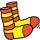# Ice cream

Annie likes much ice cream. In the shop are six kinds of ice cream. In how many ways she can buy ice cream to three scoop if each have a different flavor mound and the order of scoops doesn't matter?

Result

n =  20

#### Solution:Leave us a comment of example and its solution (i.e. if it is still somewhat unclear...):

Showing 0 comments:Be the first to comment!#### To solve this example are needed these knowledge from mathematics:

Would you like to compute count of combinations?

## Next similar examples:

1. ConfectioneryThe village markets have 5 kinds of sweets, one weighs 31 grams. How many different ways a customer can buy 1.519 kg sweets.
2. MenuOn the menu are 12 kinds of meal. How many ways can we choose four different meals into the daily menu?
3. A studentA student is to answer 8 out of 10 questions on the exam. a) find the number n of ways the student can choose 8 out of 10 questions b) find n if the student must answer the first three questions c) How many if he must answer at least 4 of the first 5 que
4. Fish tankA fish tank at a pet store has 8 zebra fish. In how many different ways can George choose 2 zebra fish to buy?
5. TeamsHow many ways can divide 16 players into two teams of 8 member?
6. ExaminationThe class is 21 students. How many ways can choose two to examination?
7. PIN - codesHow many five-digit PIN - code can we create using the even numbers?
8. BlocksThere are 9 interactive basic building blocks of an organization. How many two-blocks combinations are there?
9. Class pairsIn a class of 34 students, including 14 boys and 20 girls. How many couples (heterosexual, boy-girl) we can create? By what formula?
10. TrinityHow many different triads can be selected from the group 43 students?
11. ChordsHow many 4-tones chords (chord = at the same time sounding different tones) is possible to play within 7 tones?
12. Volleyball8 girls wants to play volleyball against boys. On the field at one time can be six players per team. How many initial teams of this girls may trainer to choose?
13. DivisionDivision has 18 members: 10 girls and 6 boys, 2 leaders. How many different patrols can be created, if one patrol is 2 boys, 3 girls and 1 leader?
14. Weekly serviceIn the class are 20 pupils. How many opportunities have the teacher if he wants choose two pupils randomly who will weeklies?
15. Theorem proveWe want to prove the sentense: If the natural number n is divisible by six, then n is divisible by three. From what assumption we started?
16. Count of trianglesGiven a square ABCD and on each side 8 internal points. Determine the number of triangles with vertices at these points.
17. Calculation of CNCalculate: ?